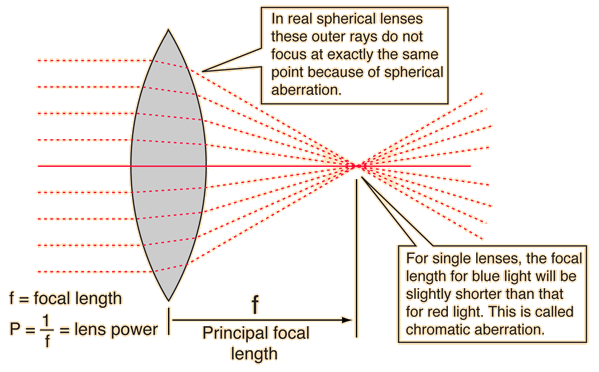# Principal Focal Length

For a thin double convex lens, refraction acts to focus all parallel rays to a point referred to as the principal focal point. The distance from the lens to that point is the principal focal length f of the lens. For a double concave lens where the rays are diverged, the principal focal length is the distance at which the back-projected rays would come together and it is given a negative sign. The lens strength in diopters is defined as the inverse of the focal length in meters. For a thick lens made from spherical surfaces, the focal distance will differ for different rays, and this change is called spherical aberration. The focal length for different wavelengths will also differ slightly, and this is called chromatic aberration.The principal focal length of a lens is determined by the index of refraction of the glass, the radii of curvature of the surfaces, and the medium in which the lens resides. It can be calculated from the lens-maker's formula for thin lenses.This shows parallel beams from two helium-neon lasers converging to the principal focal point of a 30 cm double convex lens. The rays then enter a diverging lens of focal length -10cm on the right. The laser beams were made visible with a spray can of artificial smoke.

Index

Lens concepts

 HyperPhysics***** Light and Vision R Nave
Go Back

# Focal Length and Lens Strength

The most important characteristic of a lens is its principal focal length, or its inverse which is called the lens strength or lens "power". Optometrists usually prescribe corrective lenses in terms of the lens power in diopters. The lens power is the inverse of the focal length in meters: the physical unit for lens power is 1/meter which is called diopter.Spherical aberration Chromatic aberration
Index

Lens concepts

 HyperPhysics***** Light and Vision R Nave
Go Back